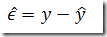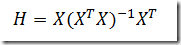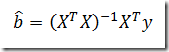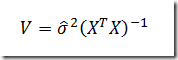## Wednesday, September 30, 2009

### Studentized residuals (the linear case)

I have developed a linear and nonlinear regression solver for GAMS (see http://www.amsterdamoptimization.com/pdf/regression.pdf and http://www.amsterdamoptimization.com/pdf/nlregression.pdf). A user asked how to get studentized residuals from these. I probably should add these to the solver so they are written to the GDX file with statistics. But first lets investigate if we can do this at the GAMS level with the current information available.

The residualsare returned in the ls.gdx file for the linear regression solver. However to calculate the standard errors of the residuals we need the (diagonals of the) hat matrix:The evaluation of this matrix needs a little bit of attention. It is known from calculating the estimatesthat the evaluation of X’X is causing lots of numerical problems. Here is an example of this for the famous Longley data set: http://yetanothermathprogrammingconsultant.blogspot.com/2008/06/demonstration-of-numerical-issues-in-ls.html. However we also export the variance-covariance matrix. This matrix is defined by:So a simple way to evaluate the Hat matrix could be:

Below is a complete GAMS example to calculate the (internally and externally) studentized residuals:

\$ontext

Regression example

Cross-section data: weekly household expenditure on food and

weekly household income from Griffiths, Hill and Judge,

1993, Table 5.2, p. 182.

Erwin Kalvelagen, october 2000

\$offtext

option decimals=8;

* make sure elements are registered in this order:
set dummy /constant,income/;

set i /i1*i40/;

table data(i, *)

expenditure income
i1        9.46       25.83
i2       10.56       34.31
i3       14.81       42.50
i4       21.71       46.75
i5       22.79       48.29
i6       18.19       48.77
i7       22.00       49.65
i8       18.12       51.94
i9       23.13       54.33
i10      19.00       54.87
i11      19.46       56.46
i12      17.83       58.83
i13      32.81       59.13
i14      22.13       60.73
i15      23.46       61.12
i16      16.81       63.10
i17      21.35       65.96
i18      14.87       66.40
i19      33.00       70.42
i20      25.19       70.48
i21      17.77       71.98
i22      22.44       72.00
i23      22.87       72.23
i24      26.52       72.23
i25      21.00       73.44
i26      37.52       74.25
i27      21.69       74.77
i28      27.40       76.33
i29      30.69       81.02
i30      19.56       81.85
i31      30.58       82.56
i32      41.12       83.33
i33      15.38       83.40
i34      17.87       91.81
i35      25.54       91.81
i36      39.00       92.96
i37      20.44       95.17
i38      30.10      101.40
i39      20.90      114.13
i40      48.71      115.46
;

variables
constant
'estimate constant term coefficient'
income
'estimate income coefficient'
sse
'sum of squared errors'
;

equations
fit(i)
'the linear model'
obj
'objective'

;

obj..     sse =n= 0;
fit(i)..  data(i,
'expenditure') =e= constant + income*data(i,'income');

option lp=ls;
model ols1 /obj,fit/;
solve ols1 minimizing sse using lp;

display constant.l, income.l, sse.l;

*
* load covariance matrix and sigma from ls.gdx
*
set k /constant,income/;
parameters
covar(k,k)
'variance-covariance matrix'
resid(i)
'residuals';
;
scalars
sigma
'variance'
'residual sum of squares'
df
'degrees of freedom'
;

*
* calculate W= inv(X'X) = COVAR/sigma^2
*
parameter W(k,k) "inv(X'X)";
alias (k,k2);
W(k,k2) = covar(k,k2)/sqr(sigma);
display W;

*
* form X
*
parameter X(i,k);
x(i,
'constant') = 1;
x(i,
'income') = data(i,'income');
display X;

*
* form H = X W X' (only diagonal)
*
parameter H(i);
h(i) =
sum(k2, sum(k, x(i,k)*w(k,k2)) * x(i,k2));
display h;

*
* (internally) studentized residuals
* this corresponds to stdres in ls.diag in R
*
parameter stud_res1(i) '(internally) studentized residuals';
stud_res1(i) = resid(i)/[sigma*sqrt(1-h(i))];
display stud_res1;

*
* (externally) studentized residuals
* this follows the Wikipedia definition
*
parameter stud_res2(i) '(externally) studentized residuals';
display stud_res2;

*
* (externally) studentized residuals
* this follows the R implementation of
* studres in ls.diag
*
parameter stud_res3(i) '(externally) studentized residuals R version';